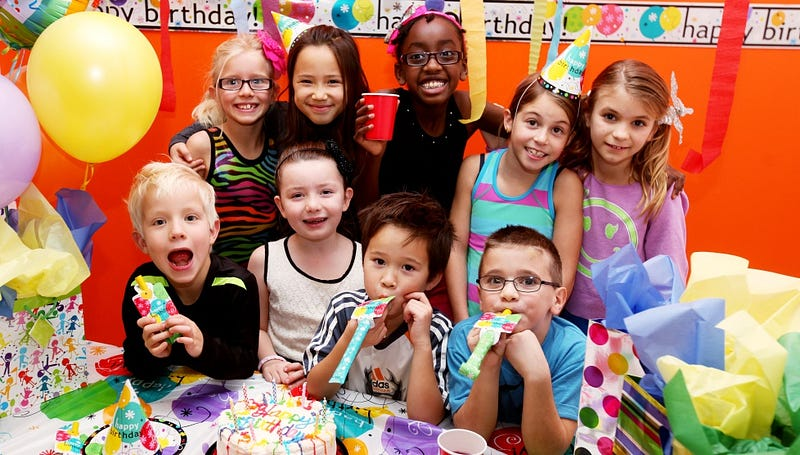Published on May 31, 2017

# Crazy Math: The Birthday Paradox

Imagine a group of people, how big do you think the group would have to be before there’s more than a 50% chance that two people in group have the same birthday.Source- http://sportcenter481.com

Assume that there are no twins and ignore leap years. Check your intuition. Take a moment to think about it. [And by same birthday, I mean same date and month of birth, Year of birth may vary].

Well, the answer may surprise you. It is surprisingly low. In a group of 23 people there is a 50.73% chance that two people will share the same birthday. But with 365 days in a year how is it possible that you need such a group to get even odds of a shared birthday. Why is our intuition so wrong? To find out the answer let’s take the help of Math.

Let’s assume P(A) is the probability that at least two people in the room have the same birthday. However, it is simpler to calculate P(A’), the probability that no two people in the room have the same birthday. Because there are only two cases that either two people have the same birthday or two people do not have the same birthday. So we can say that P(A)+P(A’) = 1.

or P(A) = 1-P(A’)

So if can calculate P(A’), the probability that no two people in the room have the same birthday, then we can easily calculate P(A), the probability that at least two people in the room have the same birthday.

Assume we have 2 persons, A and B. Person A will have one day out of 365 days as her birthday which leaves only 364 days for Person B. So the probability of A and B having different birthdays, P(A’) will be 364/365 = 0.997 or 99.7%. Assume now we have 3 persons A, B and C. We can conclude that 363 days are left for C. So the probability of A, B and C having different birthdays, P(A’) will be (364/365)*(363/365) = 0.994 or 99.4%. Assume now we have 4 persons A, B, C and D. We can again conclude that 362 days are left for D. So the probability of A, B, C and D having different birthdays, P(A’) will be (364/365)*(363/365)*(362*365) = 0.991 or 99.1%. Now if we do this for 23 persons A,B,C…W. We will get P(A’) = (364/365)*(363/365)*(362*365)…(343*365) = 0.4927 or 49.27%. So the probability of 23 persons, in which at least 2 persons having same birthday, P(A) is 100%-49.27% = 50.73%.

This P(A) is a non-linear function. As number of persons in a group grows, the probability of having same birthday gets bigger much faster.

In a group of 70 people there is a 99.9% chance that two people will share the same birthday.

Our brain is bad at grasping non-linear functions. Which is why our intuition was wrong and the answer seemed impossible at first. But once we understand the Math behind it, It starts making sense.

Thank you for your time.

Originally published on Medium By Priyansh Rastogi on May 31, 2017.